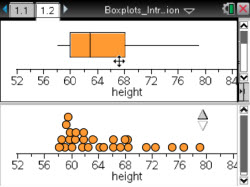Activities

••• Subject Area

• Math: Statistics: Displaying and Describing Univariate Data

• Author9-12

45 Minutes

• Device
•TI-Nspire™ CX/CX II
•TI-Nspire™ CX CAS/CX II CAS
• TI-Nspire™ Navigator™
• TI-Nspire™
• TI-Nspire™ CAS
• Software

TI-Nspire™
TI-Nspire™ CAS

3.0

Box Plots IntroductionActivity Overview

This lesson involves representing distributions of data using box plots. The emphasis is on helping students understand the relationship between individual data values and the five-number summary. Students will move data within a dot plot and observe the changes within the corresponding box plot and identify particular actions that most directly affect the five-number summary. As a result, students will develop the reasoning skills to recognize properties of a distribution from its box plot.

Objectives

• Students will describe the overall pattern of a distribution by its shape, center, and spread.
• Students will recognize and identify properties of distributions such as symmetry, skewness, and bi-modality from boxplots.
• Students will identify outliers.
• Students will recognize that moving data past a quartile can affect that quartile’s value.
• Students will reason abstractly and quantitatively (CCSS Mathematical Practice).

Vocabulary

• boxplot
• inter-quartile range
• outlier
• skewness
• interval
• range
• symmetry
• distribution
• median
• quartile